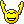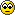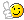Doubts in tcpdump for GCIA.

Hello Guys,

I have some queries in tcpdump as follows:

1. What filter would you use to find an IP Datagram that is greater than 40 bytes in total length?

a) ip[2:2] > 0x28
b) ip > 0x28
c) ip > 0x40
d) ip[2:2] > 0x40

Ans) a -- How do we get this answer? Please let me know how to calculate it.

2. To check if both the SYN and ACK Flags are set, use the filter of tcp=18. (True or False)

Ans) True --- How is it true?? If tcp=18 then ACK and PSH flags should be set right??

Thanks a lot for helping me.Suparn

• Posts: 1,706Member ■■■■■■■■■■
1. The field which represents the total length of the packet (including the IP header) is located at byte offset 2 in the IP header. It is also two bytes in length, and tcpdump filters will automatically assume only 1 byte of view from the offset starting point unless specified otherwise. Therefore the using ip[2:2] is needed. Since we're talking "greater than 40 bytes" and the "greater than" symbol (>) is used to represent that, we just need a hex value representing 40. This is 0x28. The "0x" says this value is in hex. The "28" represents the "40" part in hex. Since hex runs in base16, the least significant bit (the 8 in this case) is always multiplied by 1 and the second least significant bit (the 2 in this case) is multiplied by 16. So (1 x 8) + (16 x 2) = 40.

2. The TCP control bits ("flags") are located at byte offset 13 in the TCP header and this field is 1 byte in length. Therefore we use tcp as a starting point. Now let's look at the order of the TCP control bits (without the leading ECN bits):

URG, ACK, PSH, RST, SYN, FIN

Let's "count" backwards from the end to the front, starting with the bit position FIN is in. FIN is in the first bit position which represents 1, SYN represents 2, RST represents 4, PSH represents 8, ACK represents 16, and URG represents 32. If only the SYN and ACK were set, that means 2 + 16 which equals 18. Notice the answer they gave didn't say tcp = 0x18. Instead it was tcp = 18. There's no leading "0x" meaning the 18 represents a decimal value, not a hex. You can choose either decimal or value in a tcpdump filter. If you wanted to do hex instead, the answer would have been tcp = 0x12.

You probably missed the "0x" and assumed this would be hex which threw you off.
Hopefully-useful stuff I've written: http://kimiushida.com/bitsandpieces/articles/
• Wow Thanks a lot docrice.
Indeed for the first answer my guess was "d" which was correct yahoooo !!!!Yes i was confused about the second answer since i calculated in Hex and hence i was getting the wrong answer. Now i learn a little bit more. koolYour answer was really good and highly descriptive.Thanks a lot and get ready to disturbed since i am going to ask lots of doubts as i learn.Once again thanks a lot and i am really happy to be a part of this forum.You guys rock• Posts: 1,706Member ■■■■■■■■■■
The answer for the first question is actually A (ip[2:2] > 0x28), not D. 0x28 is 40 decimal. You can always use a calculator to convert between decimal and hex to confirm your answers.
Hopefully-useful stuff I've written: http://kimiushida.com/bitsandpieces/articles/
• ohh all right thanks.